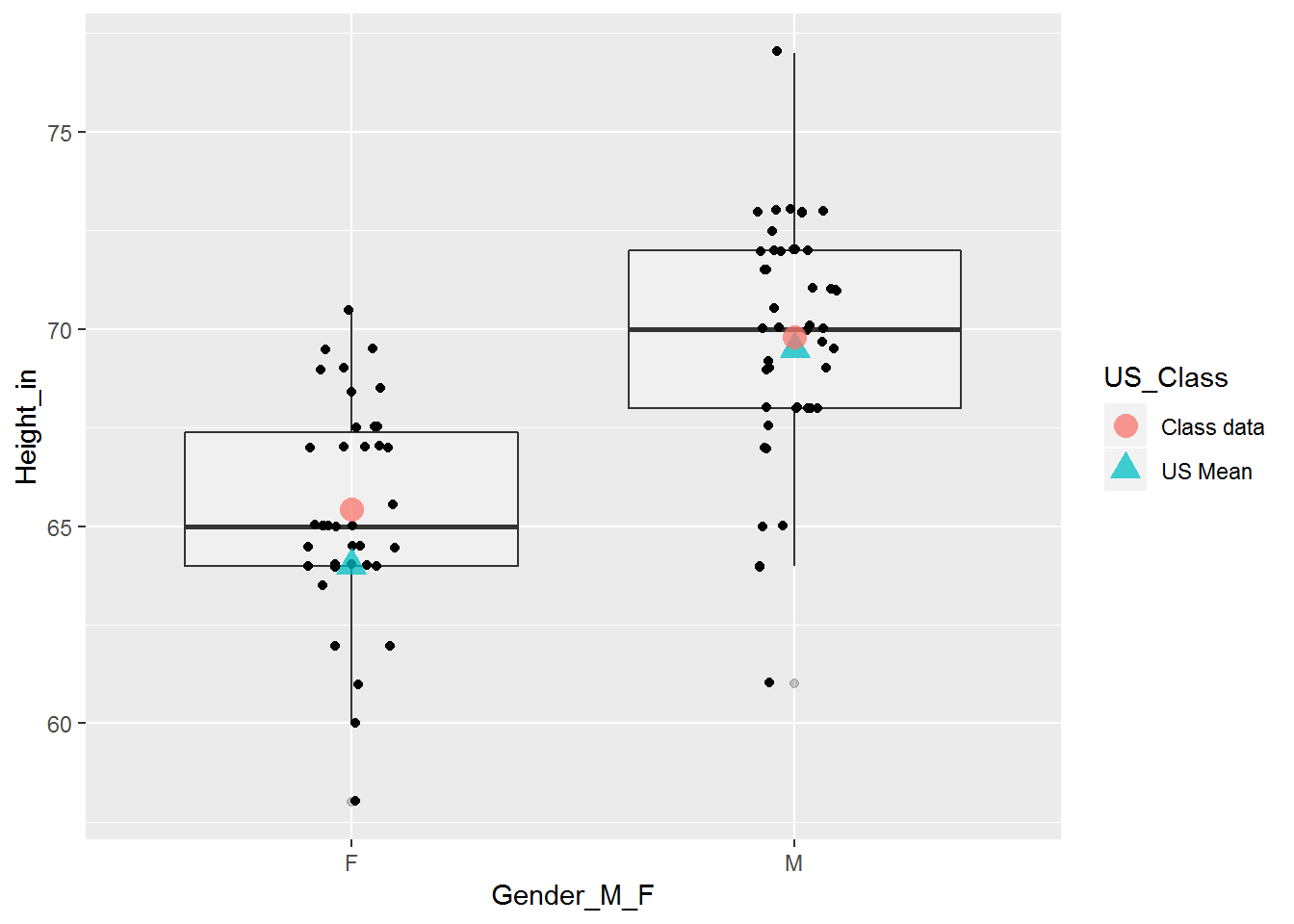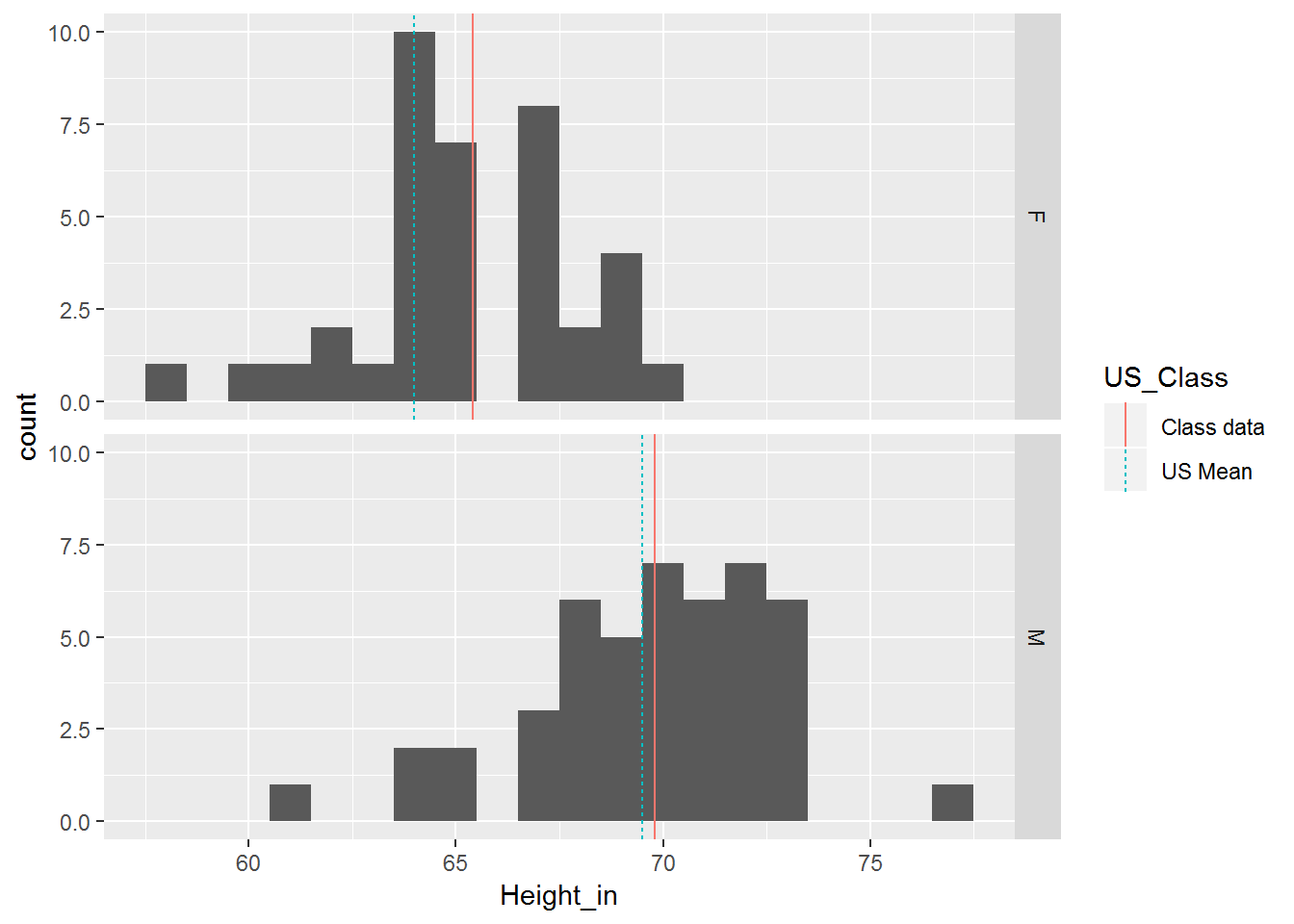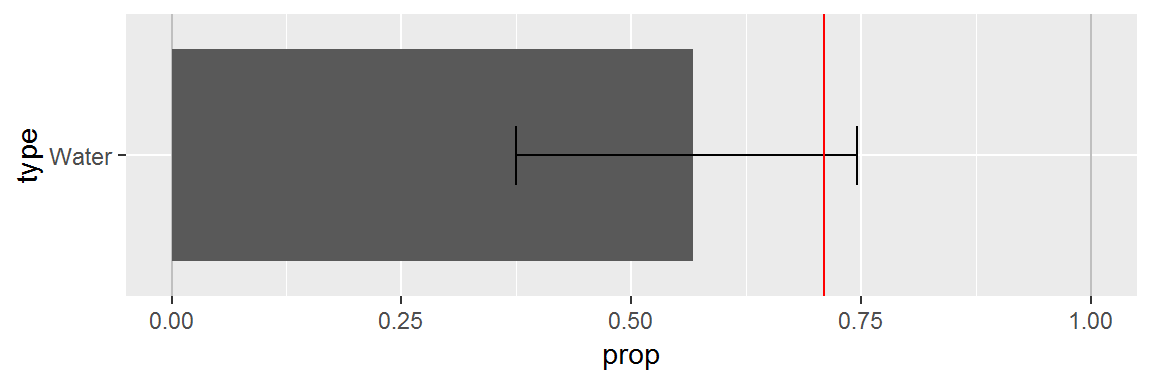Include your answers in this document in the sections below the rubric.

# Rubric

Answer the questions with the data examples.

# Mechanics of a hypothesis test (review)

1. Set up the null and alternative hypotheses in words and notation.
• In words: The population mean for [what is being studied] is different from [value of $$\mu_0$$].’’ (Note that the statement in words is in terms of the alternative hypothesis.)
• In notation: $$H_0: \mu=\mu_0$$ versus $$H_A: \mu \ne \mu_0$$ (where $$\mu_0$$ is specified by the context of the problem).
2. Choose the significance level of the test, such as $$\alpha=0.05$$.

3. Compute the test statistic, such as $$t_{s} = \frac{\bar{Y}-\mu_0}{SE_{\bar{Y}}}$$, where $$SE_{\bar{Y}}=s/\sqrt{n}$$ is the standard error.

4. Determine the tail(s) of the sampling distribution where the $$p$$-value from the test statistic will be calculated (for example, both tails, right tail, or left tail). (Historically, we would compare the observed test statistic, $$t_{s}$$, with the critical value $$t_{\textrm{crit}}=t_{\alpha/2}$$ in the direction of the alternative hypothesis from the $$t$$-distribution table with degrees of freedom $$df = n-1$$.)

5. State the conclusion in terms of the problem.
• Reject $$H_0$$ in favor of $$H_A$$ if $$p\textrm{-value} < \alpha$$.
• Fail to reject $$H_0$$ if $$p\textrm{-value} \ge \alpha$$. (Note: We DO NOT accept $$H_0$$.)
6. Check assumptions of the test (for now we skip this).

# One-sample tests

## Mean: Height data for the ADA1 F16 class

Is the population mean height of UNM students eligible to take Stat 427/527 different from the US average for men (5 ft 9 1/2 in) or women (5 ft 4 in)?

library(tidyverse)
## -- Attaching packages ---------------------------------- tidyverse 1.2.1 --
## v ggplot2 3.1.0       v purrr   0.3.0
## v tibble  2.0.1       v dplyr   0.8.0.1
## v tidyr   0.8.2       v stringr 1.4.0
## v readr   1.3.1       v forcats 0.4.0
## -- Conflicts ------------------------------------- tidyverse_conflicts() --
## x dplyr::lag()    masks stats::lag()
library(readr)

# Height vs Hand Span (ADA1 F16)
dat.hand <-
read_csv("https://statacumen.com/teach/ADA1/worksheet/ADA1_WS_11_Correlation_CorrHandSpan_F16.csv")
## Parsed with column specification:
## cols(
##   Table = col_double(),
##   Person = col_double(),
##   Gender_M_F = col_character(),
##   Height_in = col_double(),
##   HandSpan_cm = col_double()
## )
# install.packages("gsheet")

dat.hand <- na.omit(dat.hand)
dat.hand$Gender_M_F <- factor(dat.hand$Gender_M_F, levels = c("F", "M"))

str(dat.hand)
## Classes 'tbl_df', 'tbl' and 'data.frame':    84 obs. of  5 variables:
##  $Table : num 1 1 1 1 1 1 2 2 2 2 ... ##$ Person     : num  1 4 5 6 7 9 1 2 3 4 ...
##  $Gender_M_F : Factor w/ 2 levels "F","M": 1 2 1 2 1 2 1 1 1 1 ... ##$ Height_in  : num  62 72 65 70 60 72.9 69.5 67 68.5 64.5 ...
##  $HandSpan_cm: num 17 22.5 19 21 18.5 22.5 19.5 20 20 20 ... ## - attr(*, "na.action")= 'omit' Named int 2 3 8 17 18 27 34 35 36 44 ... ## ..- attr(*, "names")= chr "2" "3" "8" "17" ... ## If we create a summary data.frame with a similar structure as our data, then we ## can annotate our plot with those summaries. # calculate the estimated mean and order M then F est.mean <- as.numeric(by(dat.hand$Height_in, dat.hand$Gender_M_F, mean)) # combine true US mean with our estimated mean height.true <- data.frame(Gender_M_F = sort(unique(dat.hand$Gender_M_F))
, Height_in = c(64, 69.5, est.mean)
, US_Class = c(rep("US Mean", 2), rep("Class data", 2)))

Plot the estimated mean from our class sample versus the true US mean. Here’s two ways to plot our data, annotating the observed and hypothesized means.

height.true
##   Gender_M_F Height_in   US_Class
## 1          F  64.00000    US Mean
## 2          M  69.50000    US Mean
## 3          F  65.41842 Class data
## 4          M  69.80217 Class data
library(ggplot2)
p <- ggplot(data = dat.hand, aes(x = Gender_M_F, y = Height_in))
p <- p + geom_boxplot(alpha = 1/4)
p <- p + geom_jitter(position = position_jitter(width = 0.1))
p <- p + geom_point(data = height.true, aes(colour = US_Class, shape = US_Class), size = 4, alpha = 3/4)
print(p)library(ggplot2)
p <- ggplot(data = dat.hand, aes(x = Height_in))
p <- p + geom_histogram(binwidth = 1)
p <- p + geom_vline(data = height.true, aes(xintercept = Height_in, colour = US_Class, linetype = US_Class))
p <- p + facet_grid(Gender_M_F ~ .)
print(p)### Conduct the hypothesis tests

# look at help for t.test
# ?t.test
# defaults include: alternative = "two.sided", conf.level = 0.95

### Test female height equal to US (illustrative example).

# test females
t.summary.F <- t.test(subset(dat.hand, Gender_M_F == "F")$Height_in , mu = 64) t.summary.F ## ## One Sample t-test ## ## data: subset(dat.hand, Gender_M_F == "F")$Height_in
## t = 3.2005, df = 37, p-value = 0.002815
## alternative hypothesis: true mean is not equal to 64
## 95 percent confidence interval:
##  64.52044 66.31640
## sample estimates:
## mean of x
##  65.41842
names(t.summary.F)
##  "statistic"   "parameter"   "p.value"     "conf.int"    "estimate"
##  "null.value"  "alternative" "method"      "data.name"

Hypothesis test

1. The population mean height for females at UNM eligible to take Stat 427/527 is different from the US population value of $$\mu_0=64$$ inches.’’
• $$H_0: \mu=64$$ versus $$H_A: \mu \ne 64$$
2. Let $$\alpha=0.05$$, the significance level of the test and the Type-I error probability if the null hypothesis is true.

3. $$t_{s} = 3.201$$.

4. $$p=0.00282$$, this is the observed significance of the test.

5. Because $$p=0.00282 < 0.05$$, we have sufficient evidence to reject $$H_0$$, concluding that the observed mean height is different than the US population mean.

### Test male height greater than US (your turn).

1. (3 p) As above, set up the hypothesis test for males, but whether UNM males are taller on average than the US population.
## You'll need to modify the statement below to correspond
## to the hypothesis you wish to test
# test males
t.summary.M <- t.test(subset(dat.hand, Gender_M_F == "M")$Height_in , mu = 0 , alternative = "two.sided") t.summary.M ## ## One Sample t-test ## ## data: subset(dat.hand, Gender_M_F == "M")$Height_in
## t = 159.82, df = 45, p-value < 2.2e-16
## alternative hypothesis: true mean is not equal to 0
## 95 percent confidence interval:
##  68.92252 70.68183
## sample estimates:
## mean of x
##  69.80217

Hypothesis test

1. ’’
• $H_0:$ versus $H_A:$
2. Let $$\alpha=0.05$$, the significance level of the test and the Type-I error probability if the null hypothesis is true.

3. $t_{s} =$.

4. $p =$, this is the observed significance of the test.

5. Because $p =$, …

## Proportion: Earth’s water example

1. (3 p) In class we sampled the beach ball and observed ?? of ?? observations were water. Conduct a hypothesis test to determine whether the proportion of water on the beach ball is different from the amount of water on the earth’s surface (71%).
## notes for prop.test() and binom.test()
# x = number of "successes"
# n = total sample size

n = 30
x = 17

dat.globe <- data.frame(type = c("Water", "Land"), freq = c(x, n - x), prop = c(x, n - x) / n)
dat.globe
##    type freq      prop
## 1 Water   17 0.5666667
## 2  Land   13 0.4333333
# binom.test() is an exact test for a binomial random variable
b.summary <- binom.test(x = x, n = n, p = 0.5, conf.level = 0.95)
b.summary
##
##  Exact binomial test
##
## data:  x and n
## number of successes = 17, number of trials = 30, p-value = 0.5847
## alternative hypothesis: true probability of success is not equal to 0.5
## 95 percent confidence interval:
##  0.3742735 0.7453925
## sample estimates:
## probability of success
##              0.5666667
library(ggplot2)
p <- ggplot(data = subset(dat.globe, type == "Water"), aes(x = type, y = prop))
p <- p + geom_hline(yintercept = c(0, 1), alpha = 1/4)
p <- p + geom_bar(stat = "identity")
p <- p + geom_errorbar(aes(min = b.summary$conf.int, max = b.summary$conf.int), width=0.25)
p <- p + geom_hline(yintercept = 0.71, colour = "red")
p <- p + scale_y_continuous(limits = c(0, 1))
p <- p + coord_flip() # flip the x and y axes for horizontal plot
print(p)Hypothesis test

1. ’’
• $H_0:$ versus $H_A:$
2. Let $$\alpha=0.05$$, the significance level of the test and the Type-I error probability if the null hypothesis is true.

3. $t_{s} =$.

4. $p =$, this is the observed significance of the test.

5. Because $p =$, …

# Two-sample tests

## Means: African countries in the UN example

Previously in class we collected data using a randomized experiment. We provided a priming number (X = 10 or 65, not actually a random number) then asked you two questions:

1. Do you think the percentage of countries represented in the United Nations that are from Africa is higher or lower than X?

2. Give your best estimate of the percentage of countries represented in the United Nations that are from Africa.

The data were compiled into a google doc which we read in below.

dat.UN.Africa <-
read_csv("https://statacumen.com/teach/S4R/worksheet/S4R_WS_16_Sampling_UN_Experiment_data.csv")
## Parsed with column specification:
## cols(
##   PrimingNumber = col_double(),
##   HighLow = col_character(),
##   UN_Percentage = col_double()
## )
dat.UN.Africa$PrimingNumber <- factor(dat.UN.Africa$PrimingNumber)
dat.UN.Africa$HighLow <- factor(dat.UN.Africa$HighLow, levels = c("L", "H"))

str(dat.UN.Africa)
## Classes 'spec_tbl_df', 'tbl_df', 'tbl' and 'data.frame': 19 obs. of  3 variables:
##  $PrimingNumber: Factor w/ 2 levels "10","65": 2 1 2 1 1 1 2 2 2 1 ... ##$ HighLow      : Factor w/ 2 levels "L","H": 1 2 1 2 2 2 1 1 1 1 ...